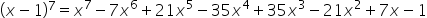Maths-
General
Easy

Question

# Use binomial theorem to expand (x - 1)7Hint:

## The correct answer is: (x + y)n

### Step 1 of 2:The given expression is (x - 1)7  , here x = x & y = -1 . The value of n=7, hence there are 7+1=8 terms in the expressions.Step 2 of 2:Substitute the values of (x - 1)7 in the binomial equation to get the expansion:Thus, the expansion is:For the expansion of an expression (x + y)n , we would have n+1 terms. This is something you need to keep in mind.#### With Turito Foundation.#### Get an Expert Advice From Turito.